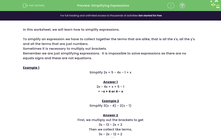# Simplifying Expressions

In this worksheet, students simplify algebraic expressions.Key stage:  KS 3

Curriculum topic:   Algebra

Curriculum subtopic:   Simplify Algebraic Expressions to Maintain Equivalence

Difficulty level:#### Worksheet Overview

In this worksheet, we will learn how to simplify expressions.

To simplify an expression we have to collect together the terms that are alike, that is all the x's, all the y's and all the terms that are just numbers.

Sometimes it is necessary to multiply out brackets.

Remember we are just simplifying expressions.  It is impossible to solve expressions as there are no equals signs and these are not equations.

Example 1

Simplify 2x + 5 - 4x - 1 + x

2x - 4x + x + 5 - 1

= -x + 4 or 4 - x

Example 2

Simplify 3(x - 4) - 2(x - 1)

First, we multiply out the brackets to get

3x - 12 - 2x + 2

Then we collect like terms.

3x - 2x - 12 + 2

x - 10

### What is EdPlace?

We're your National Curriculum aligned online education content provider helping each child succeed in English, maths and science from year 1 to GCSE. With an EdPlace account you’ll be able to track and measure progress, helping each child achieve their best. We build confidence and attainment by personalising each child’s learning at a level that suits them.

Get started